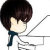0+0+0+0+0+0+0+0+0+0+0+0+0+0+0+0+0+0+0+0+0+0+0+0+Clustering with variable selection is a challenging but critical task for modern small-n-large-p data. Existing methods based on Gaussian mixture models or sparse K-means provide solutions to continuous data. With the prevalence of RNA-seq technology and lack of count data modeling for clustering, the current practice is to normalize count expression data into continuous measures and apply existing models with Gaussian assumption. In this paper, we develop a negative binomial mixture model with gene regularization to cluster samples (small \$n\$) with high-dimensional gene features (large \$p\$). EM algorithm and Bayesian information criterion are used for inference and determining tuning parameters. The method is compared with sparse Gaussian mixture model and sparse K-means using extensive simulations and two real transcriptomic applications in breast cancer and rat brain studies. The result shows superior performance of the proposed count data model in clustering accuracy, feature selection and biological interpretation by pathway enrichment analysis.0+0+0+下载预览0+0+0+0+0+0+0+0+0+0+0+0+0+0+0+0+0+0+
Top